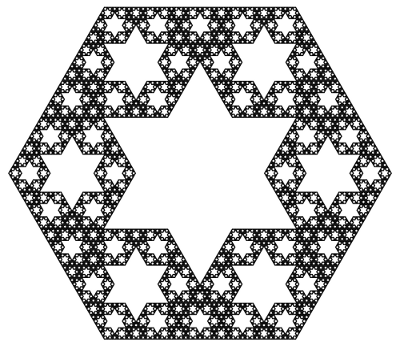# Seeing Stars

Let us now take a closer look at the hex-fractal we sliced last week. Chopping a level 0, 1, 2, and 3 Menger sponge through our slanted plane gives the following:

This suggests an iterative recipe to generate the hex-fractal. Any time we see a hexagon, chop it into six smaller hexagons and six triangles as illustrated below. Similarly, any time we see a triangle, chop it into a hexagon and three triangles like this:A recipe for constructing the hex-fractal: replace each hexagon with 6 hexagons and 6 triangles, and replace each triangle with 1 hexagon and 3 triangles.

In the limit, each triangle and hexagon in the above image becomes a hex-fractal or a tri-fractal, respectively. The final hex-fractal looks something like this (click for larger image):Now we are in a position to answer last week’s question: how can we compute the Hausdorff dimension of the hex-fractal? Let d be its dimension. Like last week, our computation will proceed by trying to compute the “d-dimensional volume” of our shape. So, start with a “large” hex-fractal and tri-fractal, each of side-length 1, and let their d-dimensional volumes be h and t respectively.

Break these into “small” hex-fractals and tri-fractals of side-length 1/3, so these have volumes $$h/3^d$$ and $$t/3^d$$ respectively (this is how “d-dimenional stuff” scales). Since $$\begin{gather*}(\text{large hex}) = 6(\text{small hex})+6(\text{small tri}) \quad \text{and}\\ (\text{large tri}) = (\text{small hex})+3(\text{small tri}),\end{gather*}$$ we find that $$h=6h/3^d + 6t/3^d$$ and $$t=h/3^d+3t/3^d$$. Surprisingly, this is enough information to solve for the value of $$3^d$$. We find $$3^d = \frac{1}{2}(9+\sqrt{33})$$, so $$d=\log_3\left(\frac{9+\sqrt{33}}{2}\right) = 1.8184\ldots,$$ as claimed last week.

As a final thought, why did we choose to slice the Menger sponge on this plane? Why not any of the (infinitely many) others? Even if we only look at planes parallel to our chosen plane, a mesmerizing pattern emerges:

It takes a bit more work to turn the above computation of the hex-fractal’s dimension into a full proof, but there are a few ways to do it. Possible methods include mass distributions or similarity graphs.

This diagonal slice through the Menger sponge has been proposed as an exhibit at the Museum of Math. Sebastien Perez Duarte seems to have been the first to slice a Menger sponge in this way (see his rendering), and his animated cross section inspired my animation above.